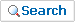<% div_no = Request("div_no") cur_page = Request.QueryString("cur_page") if cur_page = "" then cur_page = 0 end If if div_no = "" then div_no = 10 end If '°Ë»ö ĆűżäĽŇ search_div = Request("search_div") search_keyword = Replace(Request("search_keyword"),"'","") Set Cmd = Server.CreateObject("ADODB.Command") Cmd.ActiveConnection = ConnOle %> <% if ev_div="" then ev_div="7" end if %>Mail From Overseas
 Free Message Board If you have any questions, or comments, we would love to hear from you use the information top of the page to contact us by e-mail Use Free Message Board as we propose otherwise We don't accepted
<% If search_div = "bd_title" Or search_div = "bd_writer" Or search_div = "bd_content" Then search_sql = " and "&search_div&" like '%"&search_keyword&"%'" search_url = "&search_div="&search_div&"&search_keyword="&search_keyword End If News_count = 20 '¸®˝şĆ® Ç×¸ń °łĽö sql = "select bd_num, bd_stat, bd_div, bd_family, bd_depth, bd_title" sql = sql&", bd_date, bd_read, bd_writer, cmt_cnt" sql = sql&" from BOARD_FREE where bd_div="&div_no&" and bd_stat = 1" sql = sql&" union all " sql = sql&" select * from ( " sql = sql&"select top "&News_count*(cur_page+1)&" bd_num, bd_stat, bd_div, bd_family, bd_depth, bd_title" sql = sql&", bd_date, bd_read, bd_writer, cmt_cnt " sql = sql&" from BOARD_FREE where bd_div="&div_no&" " sql = sql&" and not bd_stat is null " sql = sql&search_sql sql = sql&" order by bd_family desc, bd_depth asc ) b " Cmd.CommandText = sql Cmd.CommandType = adCmdText Set rs = Cmd.Execute for count = 0 to News_count*cur_page-1 if not rs.EOF then rs.movenext end if next For counter = 0 To News_count-1 if not rs.EOF then bd_num = rs("bd_num") bd_div = rs("bd_div") bd_stat = rs("bd_stat") bd_family = rs("bd_family") bd_depth = rs("bd_depth") bd_title = rs("bd_title") bd_date = rs("bd_date") bd_read = rs("bd_read") bd_writer = rs("bd_writer") cmt_cnt = rs("cmt_cnt") ' response.write cmt_cnt Y = Mid(bd_date,1,4) M = Mid(bd_date,6,2) D = Mid(bd_date,9,2) If cmt_cnt = 0 Then cmt_cnt = "" Else cmt_cnt = "["&cmt_cnt&"]" End If %> <% rs.MoveNext end if Next rs.close %>
 No Writer Game Date Hits <%=bd_family%> <%=bd_writer%> <% If bd_depth <> chr(32) Then response.write "" end if response.write "" if bd_stat then response.write "" end if if len(bd_title) > 85 then bd_title = Left(bd_title,75) &"..." end if response.write bd_title if bd_stat then response.write "" end if response.write "" response.write ""&cmt_cnt&"" %> <%=Y&"."&M&"."&D %> <%=bd_read%>
 >Title >WriteWrite
<% sql = "select count(*)+1 " sql = sql&"from BOARD_FREE where bd_div="&div_no&" and not bd_stat is null "&search_sql Cmd.CommandText = sql Cmd.CommandType = adCmdText Set rs = Cmd.Execute if not rs.eof then total = rs(0) else total = 0 end if page = (total-1)/News_count page = Int(page) Dim i i = int(cur_page/10) * 10 if i > 1 then response.write "Start " response.write "Previous 10 " else response.write "Start " response.write "Previous 10 " End if response.write "|" for j = i to i+9 if j =< page then if j = CInt(cur_page) Then response.write ""&j+1&"|" else response.write ""&j+1&"|" end if end if Next if i+9 < CInt(page) then response.write "  Next 10 " response.write "" response.write "Last " response.write "" else response.write " Next 10" response.write "Last" end if %>